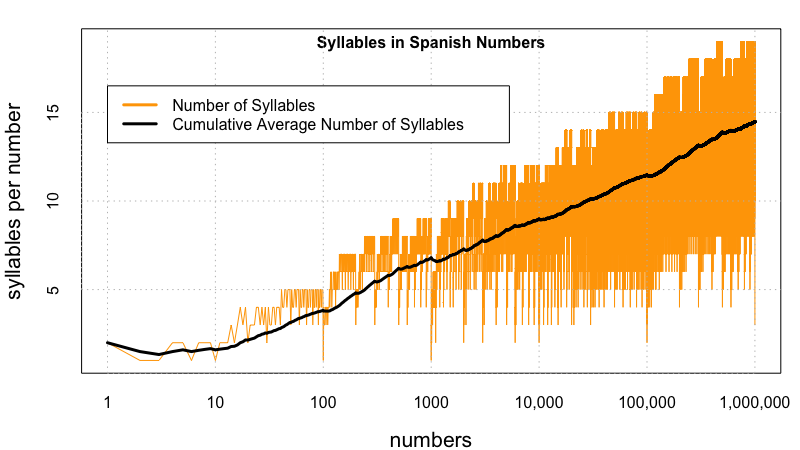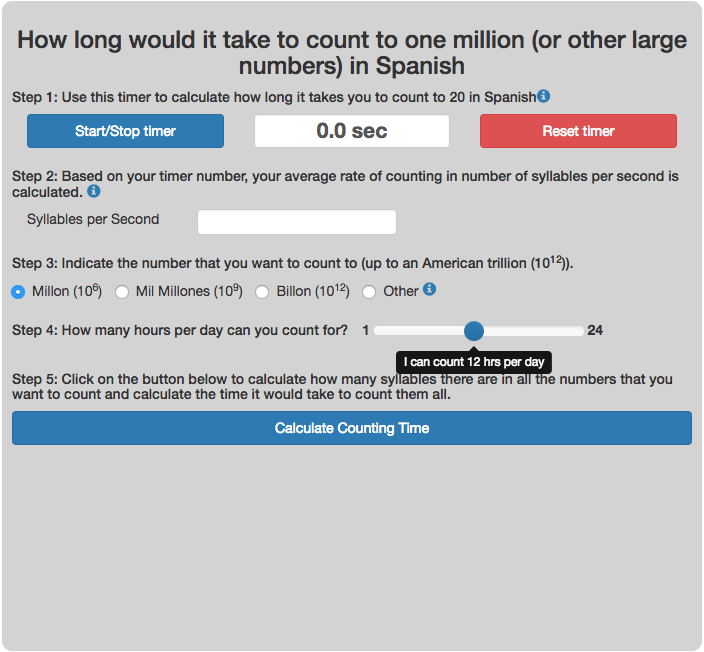# Counting to One Million, One Billion or One Trillion in Spanish

Posted In: Counting | Programming

There was lots of interest in the calculator to estimate counting time (in English) to one million, one billion and up to one trillion.  I decided to do the same for other popular languages (Spanish).  Here is the calculator that will calculate how long it takes to count to one million (or larger numbers) in Spanish.  If you’d like to see this in Spanish click here.

If you want to audit the algorithm that is used to calculate the number of syllables for counting, you can type the number in the app below to see the numeral and the calculated number of syllables.

When you plot the syllables for all the numbers from one to one million you get the following graph.  It also includes the cumulative average syllables (which reaches 14.5 syllables when averaging all numbers between 1 and 1,000,000). The maximum number of syllables in numbers between one and one million is 19 and there are lots of numbers that have this many syllables (note the log-scale axis). The first number to have that many syllables is 444,441 (cuatrocientos cuarenta y cuatro mil cuatrocientos cuarenta y uno).This histogram rearranges the data from above into bins to show the distribution of syllables in all the numbers up to one million.

For example, I say siete as “see-eh-teh” and not “sie-teh”. Anyway, if one were to count this incorrect way, it takes way longer to count to one million (about 28% longer) as my original calculation before being corrected was that the average from one to one million is 18.5 syllables per number instead of an average of 14.5.

Number Correct Syllables American Syllables
seis (6) 1 2
siete (7) 2 3
nueve (9) 2 3
diez (10) 1 2
dieciseis (16) 3 5
veintiuno (21) 3 4
ciento (100) 2 3Thanks to all the helpful Redditors in the r/Spanish and r/Linguistics groups who helped me.

Data sources and tools: Lots of web searches to better understand the numbering system for Spanish.  This info was used to create a program that could calculate the number of syllables for any number between one and 1 trillion in Javascript.  The calculator is programmed in javascript. I used R (R Studio) to make static plot to embed in this post.  The histogram is made using the open source plot.ly javascript engine.